# Flatening out a cone## Recommended PostsI'm working on a building a helmet, and I have completed a basic 3d model to help me visualize how I will build it. But I don't know how to flatten out a cone.

the helm is made out of 5 pieces, a top, a top side piece and a bottom side(the sides are fabricated twice to make a full helm)

I have a problem with the top sides and making them a flat layout.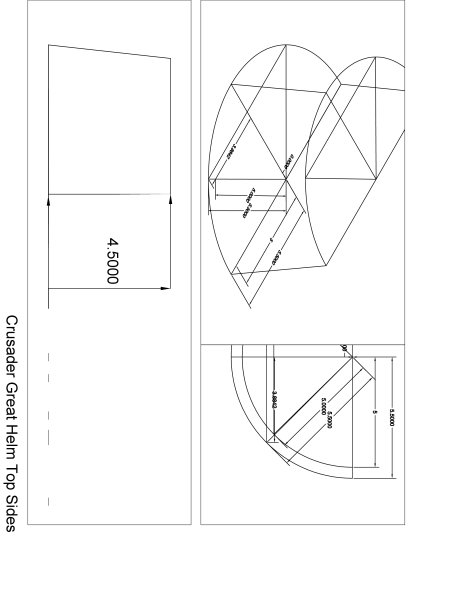##### Share on other sitesRegards

Jochen

http://www.ant-ares.de

##### Share on other sites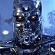I think you are basically referring to a development or pattern of a cone. You should be able to find the technique by which this is done either online or in any good technical drafting book.

Take a look at this...https://owlcation.com/stem/How-to-Develop-a-Cone

Don't cheat and take the easy way out offered above. Lay the pattern out yourself. Everyone who does drafting / design should create at least one development or pattern on their own.

##### Share on other sitesThis may be of help.

CONE.dwg

##### Share on other sites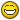CGH_TopSide.dwg

*note* I first made it a 3d model so I could visualize it.

##### Share on other sitesthis is my solution.

ReMark is right - of course. The unrolling of the cones surface can be done manually.

But if there are holes in it or you have other than simple 3d geometry, you can get in troubles...

Regards

Jochen

ant-ares.de

CGH_TopSide_scj.dwg

##### Share on other sites[ATTACH]60762[/ATTACH]

*note* I first made it a 3d model so I could visualize it.

I don't think it quite meets the definition of a 3D model. Might I suggest that you truly model the entire helmet in 3D (as a solid) so you can see how everything fits together and work out any problems with, for example, openings and attachments, before you jump right into creating templates for fabricating it? Wouldn't that make more sense?

Edited by ReMark
##### Share on other sites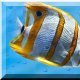```(defun C:DEVCON1 ()
;==============================================================================
;             PROGRAMME PRINCIPALE ( DEVELOPPEMENT D'UN CONE )
;
; VARIABLES UTILISEE... INVP, IVVG
; NOTE: LE PROGRAMME CALCULE (PAR DEFAULT) LES DIMENSIONS DEVELOPPEES A PARTIR
;       DE LA COTATION EXTERIEURE DU CONE.  SI LA COTATION EST DIFFERENTE, C'EST
;       ALORS QU'INTERVIENNENT LES VARRIABLES INVG ET INVP
;
; INVP=1  LORSQUE LE PETIT DIAMETRE EST COTE A L'EXTERIEUR
; INVG=1  LORSQUE LE GRAND DIAMETRE EST COTE A L'EXTERIEUR
; INVP=-1 LORSQUE LE PETIT DIAMETRE EST COTE A L'INTERIEUR
; INVG=-1 LORSQUE LE GRAND DIAMETRE EST COTE A L'INTERIEUR
;==============================================================================
(IDOD "\nSMALL DIAMETER IS INTERIOR <i> OR EXTERIOR <E> ? ")
(IF (OR (= ANS "e") (= ANS "E"))
(PROGN (SETQ INVP 1)
(SETQ PRE (/ (GETREAL "\nSMALL EXTERIOR DIAMETER=...? ") 2))
)
(PROGN (SETQ INVP -1)
(SETQ PRE (/ (GETREAL "\nSMALL INTERIOR DIAMETER =...? ") 2))
)
);FIN DU IF
(IDOD "\nLARGE DIAMETER IS INTERIOR <i> OR EXTERIOR <E> ? ")
(IF (OR (= ANS "e") (= ANS "E"))
(PROGN (SETQ INVG 1)
(SETQ GRE (/ (GETREAL "\nLARGE INTERIOR DIAMETER =...? ") 2))
)
(PROGN (SETQ INVG -1)
(SETQ GRE (/ (GETREAL "\nLARGE EXTERIOR DIAMETER =...? ") 2))
)
);FIN DU IF
(SETQ H (GETREAL "\nCONE HEIGHT =...? ")
EP (GETREAL"\nPLATE THICKNESS =...? ")
NSEG (GETREAL"\nHOW MANY SEGMENTS =...? ")
)
(DEV)     ;FONCTION DE CALCUL ET DE DESSIN DU DEVELOPPEMENT
(DIMCON)  ;FONCTION DE DESSIN DES DIMENSIONS FINI DU CONE
)          ;FIN DE LA FONCTION DEVCON

(DEFUN DIMCON (/ PTW1 PTW2 F PH3 PH4)
(SETQ  GRE (* 0.6 GRE) ; REDUCTION DE L'ECHELLE DE 'DIMCON'
PRE (* 0.6 PRE) ; MEME
H   (* 0.6 H)   ; MEME
EP  (* 0.6 EP)  ; MEME
DX  (* FACT 1.5)  ;POSITION HORIZONTALE DE  'DIMCON'
DY  (* FACT -4)   ;POSITION VERTICALE DE 'DIMCON'
)
(IF (AND (= 1 INVG) (= 1 INVP))
(POINTEX)
(IF (AND (= -1 INVG) (= -1 INVP))
(POINTIN)
(IF (AND (= 1 INVG) (= -1 INVP))
(POINTINPEXG)
(POINTEXPING)
);FIN DU IF 3
);FIN DU IF 2
);FIN DU IF 1

(SETQ PTW2 (LIST  (+ (CAR PT1) 2.5) (- (CADR PT4) 2.5))
PTW1 (LIST (- (CAR PT5) 2.5) (+ (CADR PT5) 2.5))
F (* FACT 0.4)  ;FACTEUR D'ESPACEMENT POUR LES HACHURES
PH3 (LIST (- (CAR PT3) (* EP (COS PHI))) (+ (CADR PT2) (* EP (SIN PHI))))
PH4 (LIST (+ (CAR PT1) (* EP (COS PHI))) (- (CADR PT4) (* EP (SIN PHI))))
)
;----------------------------------------------------------------------------
;PTW1 PTW2 POINTS DE REPERE POUR LA SELECTION D'OBJET LORS DES HACHURES
;PH3 PH4   POINTS DE REPERE POUR LA SELECTION D'OBJET LORS DE LA COMMANDE "MIRROR"
;F         FACTEUR D'ESPACEMENT DES HACHURES
;-----------------------------------------------------------------------------

(IF (> (* INVG INVP) 0)
(COMMAND "PLINE" PT1  "W" "0" "" PT2 PT3 PT4 "C")
(COMMAND "PLINE" PT1  "W" "0" "" PT4 PT2 PT3 "C")
)
(COMMAND "LINE" PT2 PT5 "")
(COMMAND "LINE" PT3 PT7 "")
(IF (= INVG 1)
(COMMAND "LINE" PT4 PT8 "")
(COMMAND "LINE" PT1 PT8 "")
)
(IF (AND (> (SIN PHI) 0.5) (< (SIN PHI) 0.866))  ; INCLINAISON DES HACHURES
(SETQ R 112.5)
(SETQ R 90)
)
(SETQ COT1 (RTOS (* 3.333 (- (CAR PT1) DX)) 5 4)
COT2 (RTOS (* 3.333 (- (CAR PT2) DX)) 5 4)
);AJUSTEMENT DE LA COTATION D— AU FACTEUR D`ECHELLE (0.7)

(COMMAND "HATCH" "ANSI32"  F R (SSGET "W" PH3 PH4) "" )
(COMMAND "MIRROR" "W" PTW1 PTW2 "" PT5 PT8 "")
(COMMAND "DIM" "HORI" PT1 (LIST (- (CAR PT1) (* GRE 2)) (CADR PT1))
(LIST (CAR PT4) (- (CADR PT4) (* FACT 0.5))) COT1)
(COMMAND "HORI" PT2 (LIST (- (CAR PT2) (* 2 PRE)) (CADR PT2))
(LIST (CAR PT2) (+ (CADR PT2) (* 0.5 FACT))) COT2)
(COMMAND "VERT" PT1 PT2 (LIST (+ (CAR PT1) (* 0.5 FACT)) (CADR PT1)) COT3)
(COMMAND "EXIT")
(COMMAND "REDRAW")
);FIN DE LA FONCTION DIMCONEX

(DEFUN DEV (/ PT1 PT2 PT3 PT4 PT5 PT6 PT7 PT8 PT9 PTC R1 R2 HE CL)
(SETQ PHIE 0.5)
(REPEAT 20     ;ITERATION POUR TROUVER LA PENTE EXACTE DU CONE
(SETQ PHI (ATAN (/ (- (- GRE (* EP 0.5 INVG (COS PHIE)))
(- PRE (* EP 0.5 INVP (COS PHIE)))) H))
PHIE PHI
)
)
(SETQ R1   (/ (- GRE (* EP 0.5 INVG (COS PHI))) (SIN PHI))
R2   (/ (- PRE (* EP 0.5 INVP (COS PHI))) (SIN PHI))
ALP  (/ (* PI (- GRE (* (COS PHI) INVG EP 0.5))) R1 NSEG)
)
;------------------------------------------------------------------------------
;            AJUSEMENT DES DIMENSIONS DE L`ECRAN
;
;    HE    HAUTEURE DE L`ECRAN
;    FACT  DIMENSION VALANT UN POUCE QUELQUE SOIT L`ECHELLE DU DESSIN
;    PTC   POSITION DU POINT CENTRALE DU DEVELOPPEMENT
;    CHOIX VARRIABLE INDIQUANT LE MODE DE COTATION
;-----------------------------------------------------------------------------
(IF (< ALP (/ PI 3))
(PROGN
(SETQ HE (* 2.5 R1 (SIN ALP))
FACT (* 0.091 HE)
PTC (LIST 0 (+ (* FACT -2) R2 (/ (- R1 R2) 2)))
CHOIX 0
)
)
)
(IF (AND (>= ALP (/ PI 3)) (<= PHI (/ PI 2)))
(PROGN
(SETQ HE (* 3 R1)
FACT (* 0.091 HE)
PTC (LIST 0 (+ (* FACT -2) (/ R1 2)))
CHOIX 0
)
)
)
(IF (> ALP (/ PI 2))
(PROGN
(SETQ HE (* 3.4 R1)
FACT (* 0.091 HE)
PTC (LIST 0 (* FACT -3))
CHOIX 1
)
)
) ;FIN DU IF

(SETQ PLIM1 (LIST (* HE -0.75) (* HE -1)) ;POINT LIMITE INFERIEUR GAUCHE
PLIM2 (LIST (* HE 0.75) (* HE 1))   ;POINT LIMITE SUPERIEUR DROIT
;----------------------------------------------------------------------------
;    POUR LA DEFINITION DES POINTS PT1 A PT9,CONSULTER LE DOCUMENT...
;----------------------------------------------------------------------------
PT1 (LIST (* R2 (SIN ALP)) (- (* R2 (COS ALP)) (CADR PTC)))
PT2 (LIST (* R1 (SIN ALP)) (- (* R1 (COS ALP)) (CADR PTC)))
PT3 (LIST (* R1 (SIN (* ALP -1))) (- (* R1 (COS ALP)) (CADR PTC)))
PT4 (LIST (* R2 (SIN (* ALP -1))) (- (* R2 (COS ALP)) (CADR PTC)))
PT5 (LIST 0 (- R1 (CADR PTC)))
PT6 (LIST 0 (- R2 (CADR PTC)))
PT7 (LIST (* R1 (SIN (/ ALP 1.5)))
(- (* R1 (COS (/ ALP 1.5))) (CADR PTC)))
PT8 (LIST (* R2 (SIN (* ALP 0.))
(- (* R2 (COS (* ALP 0.)) (CADR PTC)))
PT9 (LIST (* R1 (SIN (/ ALP -4)))
(- (* R1 (COS (/ ALP -4))) (CADR PTC)))
)
;-----------------------------------------------------------------------------
;                      COMMANDES DE DESSIN...
;-----------------------------------------------------------------------------
(COMMAND "LIMITS" PLIM1 PLIM2)
(COMMAND "ZOOM" "ALL")
(COMMAND "LINE" PT1 PT2 "")
(COMMAND "LINE" PT4 PT3 "")
(COMMAND "ARC" PT2 PT5 PT3)
(COMMAND "ARC" PT1 PT6 PT4)
(SETVAR "DIMTIX" 0)              ;PLACE LES DIM A L'INTERIEUR
(SETVAR "DIMSCALE" (* 1.2 FACT)) ;AJUSTE LA DIMENSION DES COTES
(SETVAR "LTSCALE" (* 0.8 FACT))  ;AJUSTE LE FACTEUR D`ECHELLE DES LIGNES
(SETVAR "LUNITS" 5 )             ;CHOIX DU SYSTEME D`UNITE FRACTIONNAIRE
(COMMAND "LINETYPE" "S" "CENTER" "")
(COMMAND "LINE" (LIST 0 (+ (CADR PT5) (* FACT 0.75)))
(LIST 0 (- (CADR PT6) (* FACT 0.75))) "")
(COMMAND "LINETYPE" "S" "CONTINUOUS" "")

(IF (= CHOIX 0)
(PROGN (SETVAR "DIMSE2" 1)      ;ENLEVE LA DEUXIEME LIGNE D`EXTENSUON
(COMMAND "DIM" "HORI" PT2 PT5 (LIST 0 (+(CADR PT5) (* 0.5 FACT)))"")
(SETVAR "DIMSE2" 0)            ;REMET LA DEUXIEME LIGNE D`EXTENSION
(COMMAND "HORI" PT1 PT2 (LIST 0 (- (CADR PT1) (* 0.5 FACT))) "")
(COMMAND "HORI" PT2 (LIST (* (CAR PT2) -1) (CADR PT2)) (LIST 0 (+ (CADR PT5) (* 1 FACT)))"")
(COMMAND "VERT" PT2 PT1 (LIST (+ (CAR PT2) (* 0.75 FACT)) 0)"")
(COMMAND "VERT" PT1 PT5 (LIST (+ (CAR PT2) (* 1.75 FACT)) 0)"")
(COMMAND "RADIUS" PT7 "" (* -1.5 FACT))
(COMMAND "RADIUS" PT8 "" (* -1.0 FACT))
(COMMAND "EXIT")
);FIN DU PROGN THEN
(PROGN
(COMMAND "DIM" "HORI" PT2 PTC (LIST 0 (- (CADR PT2) (* 0.5 FACT)))"")
(COMMAND "VERT" PT2 PTC (LIST (+ R1 (* 0.5 FACT)) 0)"")
(COMMAND "RADIUS" PT9 "" (* -2 FACT))   ;COTATION GRAND RAYON
(COMMAND "RADIUS" PT8 "" (* -0.8 FACT)) ;COTATION PETIT RAYON
(COMMAND "EXIT")
);FIN DU PROGN ELSE
);FIN DU IF

(COMMAND "REGENALL")
);FIN DE LA FONC. DEVCON

(DEFUN IDOD (ARG)
(INITGET 1 "I i E e")
(SETQ ANS (STRCASE (GETKWORD ARG)))
)

;===========================================================================
;LES 4 FONCTION SUIVANTES, CALCULENT LES POINTS POUR LE DESSIN DU CONE FORME
;===========================================================================

(DEFUN POINTEX ()
;---------------------------------------------------------------------------
;  - FONCTION UTILISEE LORSQUE LA COTATION EST EXTERIEUR (LES 2 DIAMETRES)
;  - POUR LA DEFINITION DES POINTS CONSULTER LE DOCUMENT...
;---------------------------------------------------------------------------
(SETQ PT1 (LIST (+ DX GRE) (+ DY (/ H -2)))
PT2 (LIST (+ DX PRE) (+ DY (/ H 2)))
PT3 (LIST (+ DX (- PRE (* EP (COS PHI)))) (+ DY (- (/ H 2) (* EP (SIN PHI)))))
PT4 (LIST (+ DX (- GRE (* EP (COS PHI)))) (+ DY (- (/ H -2) (* EP (SIN PHI)))))
PT5 (LIST DX (+ DY (/ H 2)))
PT7 (LIST DX (+ DY (- (/ H 2) (* EP (SIN PHI)))))
PT8 (LIST DX (+ DY (- (/ H -2) (* EP (SIN PHI)))))
)
)
(DEFUN POINTIN ()
;---------------------------------------------------------------------------
;  - FONCTION UTILISEE LORSQUE LA COTATION EST INTERIEUR (LES 2 DIAMETRES)
;  - POUR LA DEFINITION DES POINTS CONSULTER LE DOCUMENT...
;---------------------------------------------------------------------------
(SETQ PT1 (LIST (+ DX GRE) (+ DY (/ H -2)))
PT2 (LIST (+ DX PRE) (+ DY (/ H 2)))
PT3 (LIST (+ DX PRE (* EP  (COS PHI))) (+ DY (/ H 2) (* EP  (SIN PHI))))
PT4 (LIST (+ DX GRE (* EP  (COS PHI))) (+ DY (/ H -2) (* EP  (SIN PHI))))
PT5 (LIST DX (+ DY (/ H 2)))
PT7 (LIST DX (+ DY (/ H 2) (* EP (SIN PHI))))
PT8 (LIST DX (+ DY (/ H -2) ))
)
)
(DEFUN POINTINPEXG ()
;---------------------------------------------------------------------------
;  - FONCTION UTILISEE LORSQUE LA COTATION EST EXTERIEURE GRAND DIA.
;                                           ET INTERIEURE PETIT DIA.
;  - POUR LA DEFINITION DES POINTS CONSULTER LE DOCUMENT...
;---------------------------------------------------------------------------
(SETQ PT1 (LIST (+ DX GRE) (+ DY (/ H -2)))
PT2 (LIST (+ DX PRE) (+ DY (- (/ H 2) (* (SIN PHI) EP))))
PT3 (LIST (+ DX PRE (* EP  (COS PHI))) (+ DY (/ H 2) ))
PT4 (LIST (+ DX (- GRE (* EP  (COS PHI)))) (+ DY (- (/ H -2) (* EP (SIN PHI)))))
PT5 (LIST DX (+ DY (- (/ H 2) (* EP (SIN PHI)))))
PT7 (LIST DX (+ DY (/ H 2) ))
PT8 (LIST DX (+ DY (- (/ H -2) (* EP (SIN PHI)))))
)
)
(DEFUN POINTEXPING ()
;---------------------------------------------------------------------------
;  - FONCTION UTILISEE LORSQUE LA COTATION EST EXTERIEUR PETIT DIA.
;                                           ET INTERIEUR GRAND DIA.
;  - POUR LA DEFINITION DES POINTS CONSULTER LE DOCUMENT...
;---------------------------------------------------------------------------
(SETQ PT1 (LIST (+ DX GRE) (+ DY (/ H -2)))
PT2 (LIST (+ DX PRE) (+ DY (* EP (SIN PHI)) (/ H 2)))
PT3 (LIST (+ DX PRE (- (* EP  (COS PHI)))) (+ DY (/ H 2)))
PT4 (LIST (+ DX GRE (* EP  (COS PHI))) (+ DY (/ H -2) (* EP  (SIN PHI))))
PT5 (LIST DX (+ DY (* EP (SIN PHI) (/ H 2))))
PT7 (LIST DX (+ DY (/ H 2)))
PT8 (LIST DX (+ DY (/ H -2)))
)
)
```

##### Share on other sites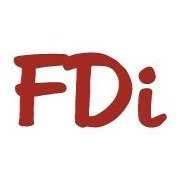If you model the piece as a legacy RULESURF mesh ( sysvar MESHTYPE=0 )

-David

## Join the conversation

You can post now and register later. If you have an account, sign in now to post with your account.
Note: Your post will require moderator approval before it will be visible.×   Pasted as rich text.   Restore formatting

Only 75 emoji are allowed.